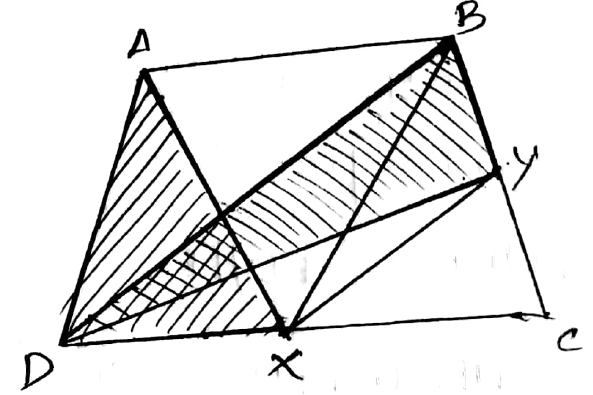Question

# ABCD is a trapezium with AB∥DC. A line parallel to BD intersects CD at X and BC at Y such that BD∥XY. Prove that ar(ADX)=ar(BDY).

Open in App
Solution

##Given ABCD is a trapezium where AB∥DC.BD intersect CD at X and BC at Y i.e, BD∥XY.We have to prove that ar(ADX)=ar(BDY)For △BDX and △BDY lie on the same base BD and are between parallel lines BD and XY.∴ar(△BDX)=ar(△BDY)[ triangle with same base and between the same parallels are equal in area. ]For △BDX and △ADX lie on the same base DX and are between parallel lies AB and DX.[ As AB∥DC, parts of parallel lines are parallel ]∴ar(△BDX)=ar(△ADX)Triangles with same base and between the same parallel are equal in area.⇒ar(△ADX)=ar(△BDY)Hence, the answer is proved.Suggest Corrections0Similar questionsRelated VideosCriteria for Similarity of Triangles_video tackle
MATHEMATICS
Watch in AppExplore more